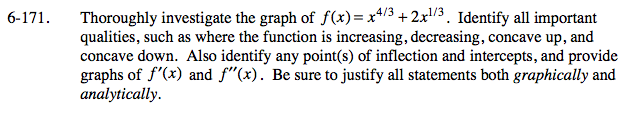### Home > CALC > Chapter 6 > Lesson 6.5.1 > Problem6-171

6-171.

Thoroughly investigate the graph of f(x) = x4/3 + 2x1/3 . Identify all important qualities, such as where the function is increasing, decreasing, concave up, and concave down. Also identify any point(s) of inflection and intercepts, and provide graphs of f ′(x) and f ″(x). Be sure to justify all statements both graphically and analytically. Homework Help ✎Remember to investigate all extrema CANDIDATES. That means, find all values of x where f'(x) = 0 AND f '(x) DNE.
After all, a max or min could exist at a cusp... But remember, sometimes these candidates turn out to be inflection points instead.

When looking for points of inflection, remember to consider where concavity changes. This could happen where f ''(x) = 0
or where f ''(x) = DNE.